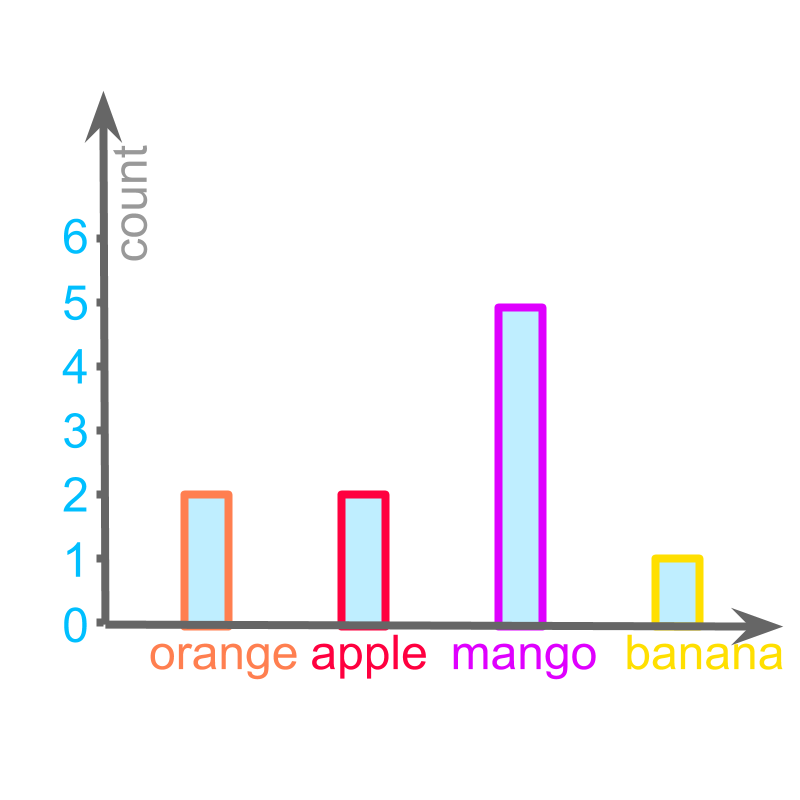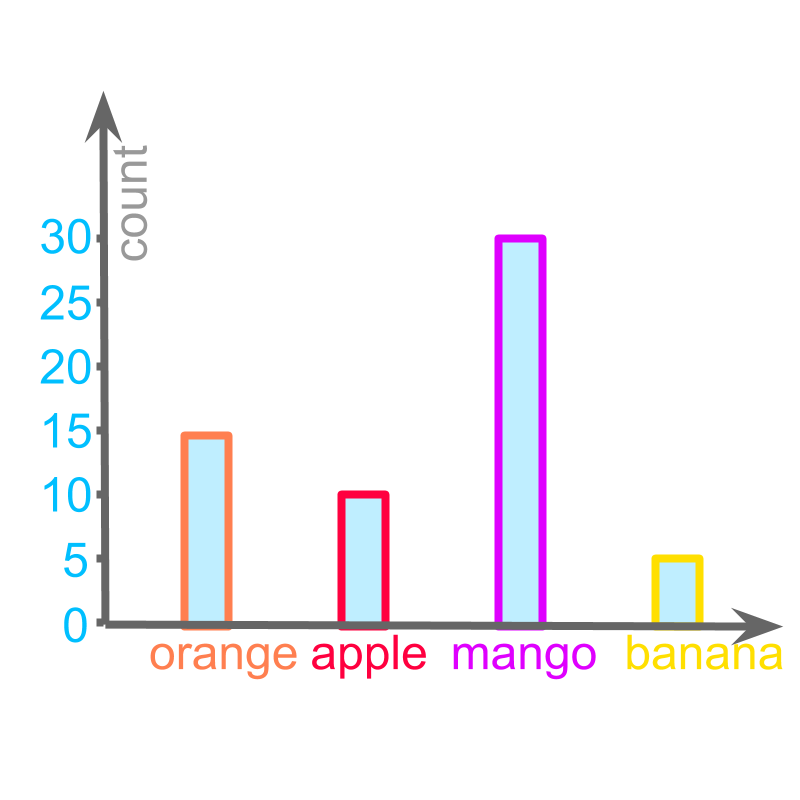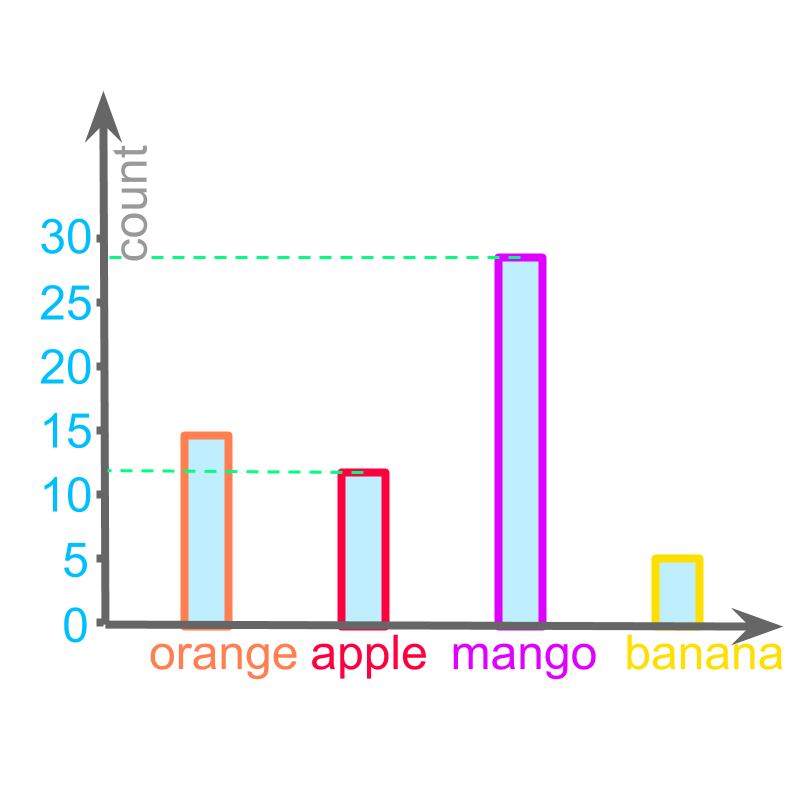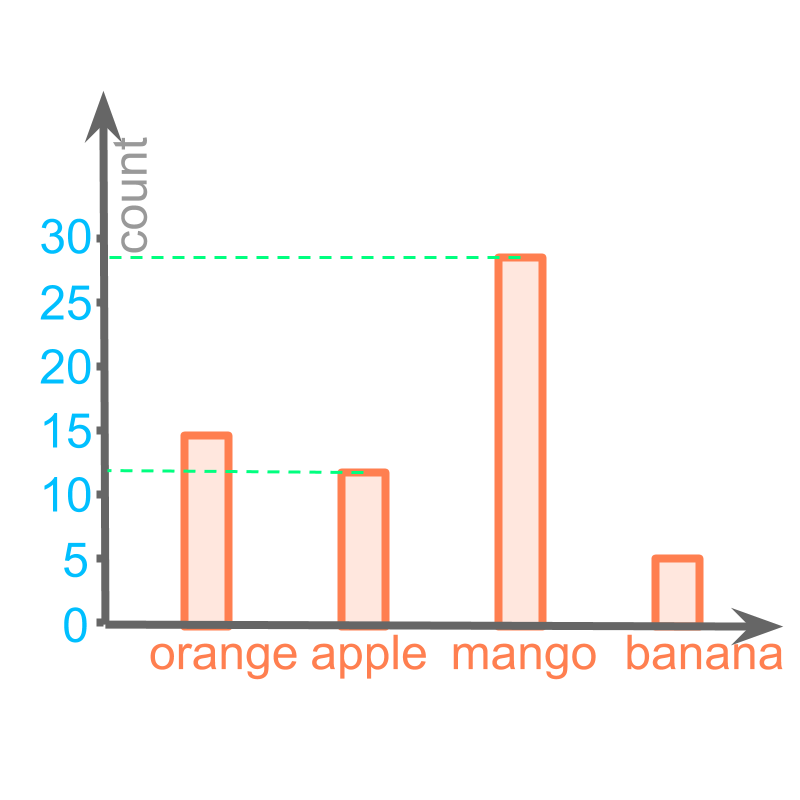maths > statistics-basics

Bar-graph representation

what you'll learn...

overview

In this page, the bar-graph representation of Data is explained with simple examples.

•  Bar graph is a spatial representation of data with bars in vertical and horizontal axis

bar

In the earlier lessons, Data representation using tally marks, table, and pictograph were explained. These representations were useful to understand details like which data-value is large, which data-values are comparable , etc.

The data can be given in a spatially laid out visual form. It is widely used in newspapers and other publications.Consider the data: Number of students who took a given fruit.

orange $2$$2$
apple $2$$2$
mango $5$$5$
banana $1$$1$

The figure depicts a "bargraph" of the data.

The word "bar-graph" means: data represented with bars. The representation can be with horizontal or vertical bars.

scaling the bar

Earlier, we learned about scaling in pictographs. Scaling was introduced to represent large data-values.

The bar graphs have a similar problem. The numbers marked in vertical axis will be too close for large values.The following data is represented:
Orange : $15$$15$
Apple : $10$$10$
Mango : $30$$30$
Banana : $5$$5$

Instead of marking each of the values in the vertical axis, the values are marked in increment of $5$$5$. This is illustrated in the figure.The number of apples $12$$12$ is represented with a vertical bar of height $12$$12$.

Similarly the number of mangoes $28$$28$ is represented with a vertical bar of height $28$$28$.

summaryBar-Graph : A spatial representation of data with bars in vertical and horizontal axis.

Outline